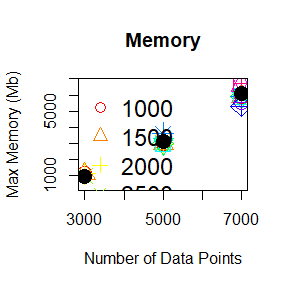# Objective

In this very synthetic tutorial, we will walk readers through different analyses and example of usages of the latest version of R package crqa() as they appear in Coco, et, al., (in press). Our goal is to provide readers with a reference guide to all analyses that are possible with crqa(). We refer readers to such a paper for greater details about the theoretical insights associated with these sets of analysis.

library(crqa)      # The crqa() package
library(pracma)    # To simulate data (sine waves) 
data(crqa) # Load the data available in the package 

## Initialise constants and variables that we will need later on.

options(scipen = 999) # Disable scientific notation

# Alpha colours for illustrating diagonal|windowed-crqa
diagcol = "#FF000080" # gray 80
windcol = "#36648B80" # gray 51

## The focus window for the text example (Figure 4)
xd = c(100, 0,   1800, 2000)
yd = c(0, 100, 2000, 2000)

xw = c(0, 0,   100, 100)
yw = c(0, 100, 100, 0)

# Methodological background

## Initialize arguments needed to produce the recurrence plots of Figure 1

parC = list(unit = 200, labelx = "", labely = "",
cols = "black", pcex = .3, pch = 20, las = 0,
labax = seq(0, nrow(Figure_1), 200), labay = seq(0, nrow(Figure_1), 200)) 

## Figure 1

### Top row

par(mfrow = c(1, 3), font.lab = 2, font.axis = 2, cex.lab = 2, cex.axis = 1.5)

plot(Figure_1$sinus, type = "l", xlab = "", ylab = "", axes = F, lwd = 2) box() plot(Figure_1$lorenz, type = "l", xlab = "", ylab = "", axes = F, lwd = 2)
box()

plot(Figure_1$wnoise, type = "l", xlab = "", ylab = "", axes = F, lwd = 2) box()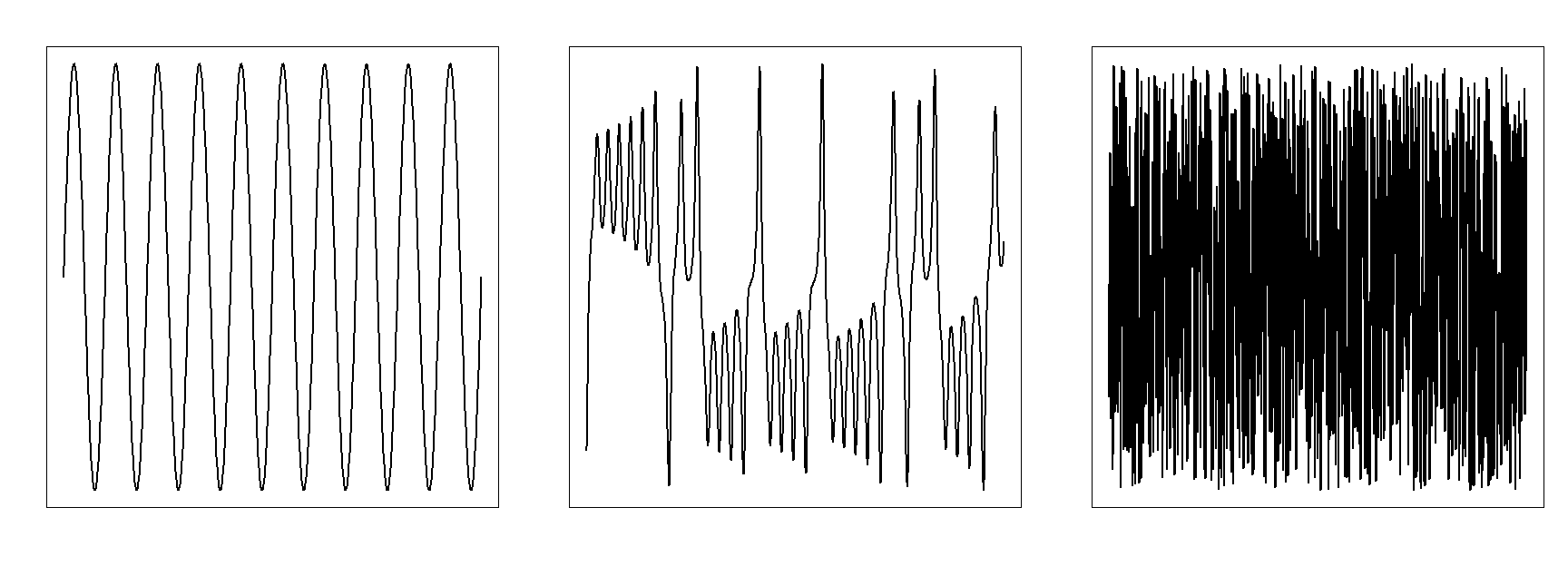### Mid row SINrqa1 <- crqa(ts1 = Figure_1$sinus, ts2 = Figure_1$sinus, delay = 1, embed = 1, rescale = 0, radius = 0.03, normalize = 0, side = 'both', method = 'rqa', datatype = 'continuous') LORrqa1 <- crqa(ts1 = Figure_1$lorenz, ts2 = Figure_1$lorenz, delay = 1, embed = 1, rescale = 0, radius = 0.03, normalize = 0, side = 'both', method = 'rqa', datatype = 'continuous') WNOISErqa1 <- crqa(ts1 = Figure_1$wnoise, ts2 = Figure_1$wnoise, delay = 1, embed = 1, rescale = 0, radius = 0.003, normalize = 0, side = 'both', method = 'rqa', datatype = 'continuous') par(mfrow = c(1,3), font.lab = 2, font.axis = 2, cex.lab = 2, cex.axis = 1.5, mar = c(1, 5.5, 3, 2), oma = c(1,1,2,1)) plotRP(SINrqa1$RP, parC)
plotRP(LORrqa1$RP, parC) plotRP(WNOISErqa1$RP, parC)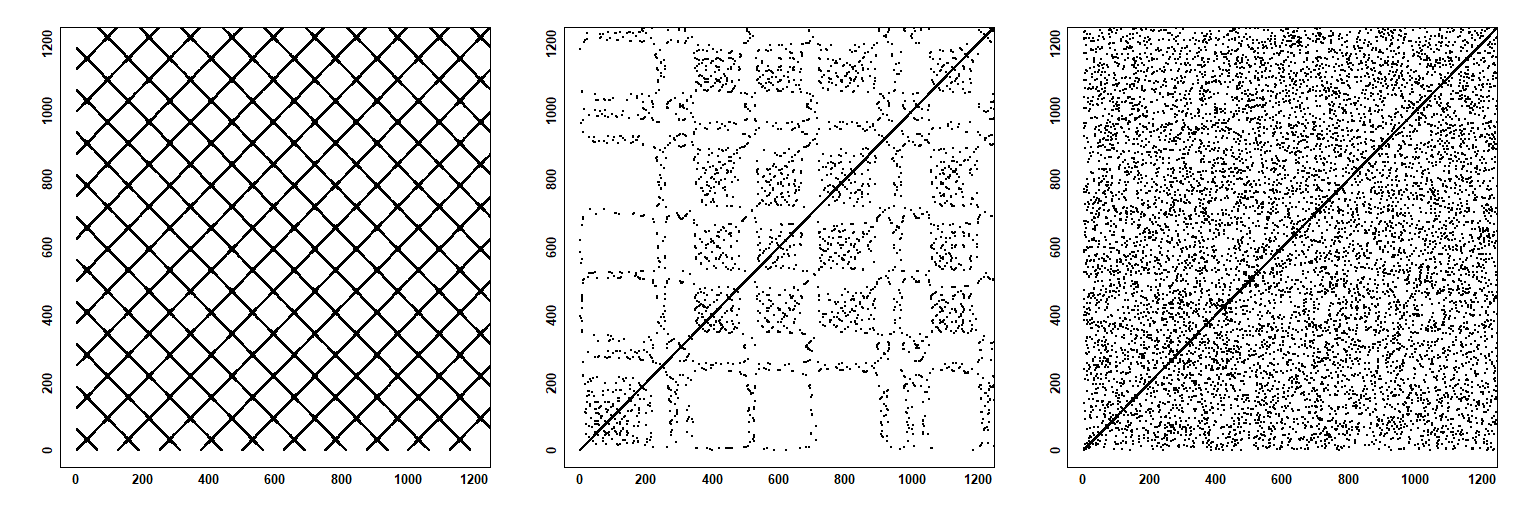### Bottom row

SINrqa2 <- crqa(ts1 = Figure_1$sinus, ts2 = Figure_1$sinus, delay = 110,
embed = 2, rescale = 0, radius = 0.02, normalize = 0,
side = 'both', method = 'rqa', datatype = 'continuous')

LORrqa2 <- crqa(ts1 = Figure_1$lorenz, ts2 = Figure_1$lorenz, delay = 10,
embed = 3, rescale = 0, radius = 1.3, normalize = 0,
side = 'both', method = 'rqa', datatype = 'continuous')

WNOISErqa2 <- crqa(ts1 = Figure_1$wnoise, ts2 = Figure_1$wnoise, delay = 1,
embed = 3, rescale = 0, radius = 0.1, normalize = 0,
side = 'both', method = 'rqa', datatype = 'continuous')

par(mfrow = c(1,3), font.lab = 2, font.axis = 2, cex.lab = 2, cex.axis = 1.5,
mar = c(1, 5.5, 3, 2), oma = c(1,1,2,1))

## The sinusoid has a longer delay, so we need to change the label to reflect this
parC$labax = seq(0, 1000, 200); parC$labay = seq(0,1000, 200)
plotRP(SINrqa2$RP, parC) parC$labax = seq(0, 1200, 200); parC$labay = seq(0,1200, 200) plotRP(LORrqa2$RP, parC)
plotRP(WNOISErqa2$RP, parC)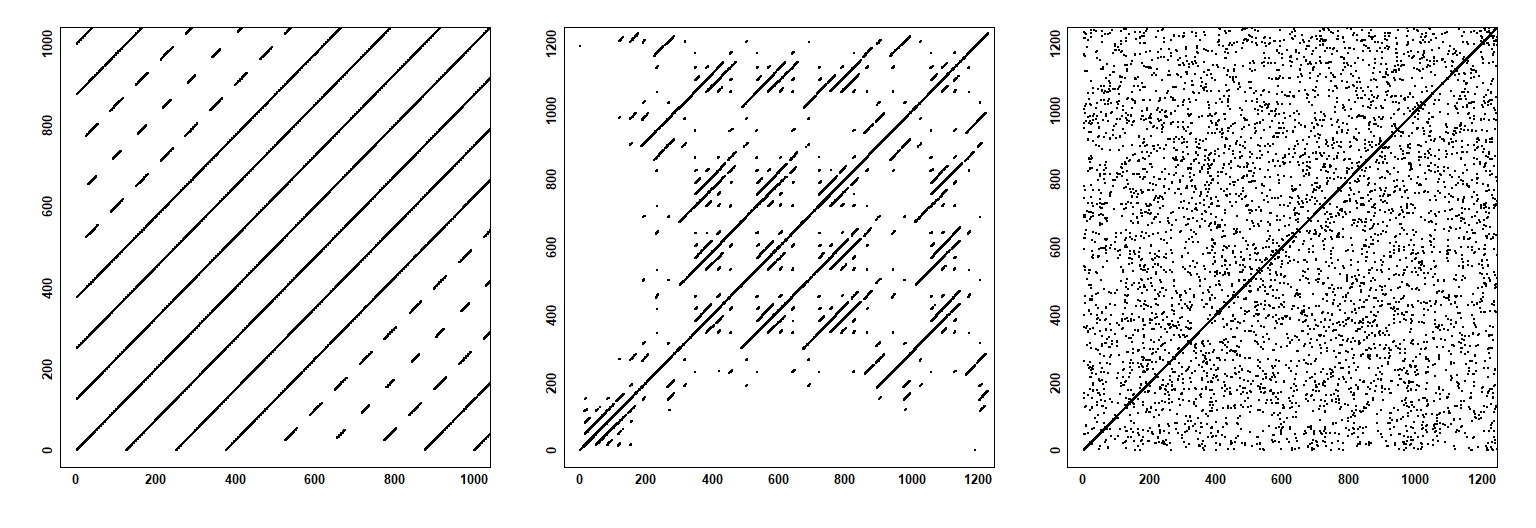## Figure 2 Figure_2 = as.numeric(as.matrix(Figure_2)) # Make it a vector ### Figure 2.A par(font.lab = 2, font.axis = 2, cex.lab = 1.7, cex.axis = 1.5) plot(Figure_2[1:42], Figure_2[4:45], type = 'b', las = 1, xlab = 'dimension 1', ylab = 'dimension 2', main = '', lwd = 2) points(Figure_2, Figure_2, pch = 19, cex = 1.2, col = "blue") points(Figure_2, Figure_2, pch = 19, cex = 1.2, col = "green") points(Figure_2, Figure_2, pch = 19, cex = 1.2, col = "red") symbols(x = Figure_2, y = Figure_2, circles = 0.25, add = TRUE, inches = FALSE) points(Figure_2, Figure_2, pch = 19, cex = 1.2, col = "red") points(Figure_2, Figure_2, pch = 19, cex = 1.2, col = "red") points(Figure_2, Figure_2, pch = 19, cex = 1.2, col = "red") text(x = -0.5, y = -1.1, labels = "Threshold", adj = 1) text(x = -0.6, y = -0.2, adj = 0, label = "Recurrences of the point") text(x = -0.6, y = -0.3, adj = 0, bquote('('~X*';'~X[9+tau]*')'))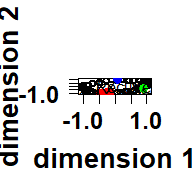### Figure 2.B par(font.lab = 2, font.axis = 2, cex.lab = 2, cex.axis = 1.5) plot(Figure_2, 45:1, type = 'b', las = 1, xlab = "", ylab = "time", yaxt = "n") points(Figure_2, 45, pch = 19, cex = 1.2, col = "blue") points(Figure_2, 40, pch = 19, cex = 1.2, col = "green") points(Figure_2, 37, pch = 19, cex = 1.2, col = "red") axis(side = 2, at = seq(from = 45, to = 5, by = -10), las = 1, labels = c(0, 10, 20, 30, 40), tick = T)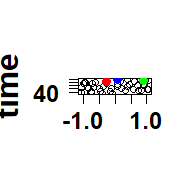### Figure 2.C par(font.lab = 2, font.axis = 2, cex.lab = 2, cex.axis = 1.5) plot(Figure_2[4:45], type = 'b', las = 1, xlab = 'time', ylab = '') points(1, Figure_2, pch = 19, cex = 1.2, col = "blue") points(6, Figure_2, pch = 19, cex = 1.2, col = "green") points(9, Figure_2, pch = 19, cex = 1.2, col = "red")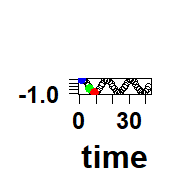### Figure 2.D fig2rqa <- crqa(ts1 = Figure_2, ts2 = Figure_2, delay = 3, embed = 2, radius = 0.25, method = 'rqa', datatype = 'continuous') parC$unit = 10; parC$labax = ""; parC$labay = ""; parC$pcex = 1; parC$pch = 15

par(font.lab = 2, font.axis = 2, cex.lab = 2, cex.axis = 1.5)

plotRP(fig2rqa$RP, parC) ixi_9 = which(fig2rqa$RP[9,] == T)
points(rep(9, length(ixi_9)), ixi_9, col = "red", pch = 15, cex = 1)
points(ixi_9, rep(9, length(ixi_9)), col = "red", pch = 15, cex = 1)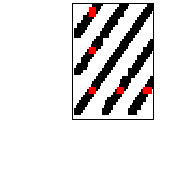## Figure 3

par(mfrow = c(1,2), font.lab = 2, font.axis = 2, cex.lab = 2, cex.axis = 1.5,
mar = c(4.5, 4.5, 1, 1))

L1 <- Figure_3[,1]
L2 <- Figure_3[,2]

# Panel A.
plot(L2, type = "l", xlab = "time", ylab = "", lwd = 2)
lines(L1, col = "red", lwd = 2)

# Panel B

# Run DCRP analysis on data
Fig3 <- drpfromts(ts1 = L2, ts2 = L1, delay = 1, embed = 1,
windowsize = 20, radius = 0.02, datatype = "continuous",
rescale = 0, normalize = 0, mindiagline = 2,
minvertline = 2, tw = 0, side = 'both', method = "crqa")

# Plot the recurrence profile
plot(-20:20,  Fig3$profile, type = "b", lwd = 2, ylab = "Recurrence Rate", xlab = "Lag", cex = 0.8) abline(v = 0, lty = 2)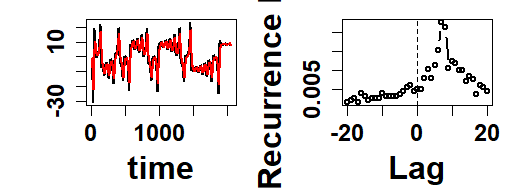# Using the crqa package ## Recurrence quantification analysis (RQA) ### Figure 4 (left panel) ## Setting the parameters delay = 1; embed = 1; rescale = 0; radius = 0.0001; normalize = 0; mindiagline = 2; minvertline = 2; tw = 1; whiteline = FALSE; recpt = FALSE; side = "both"; method = 'rqa'; metric = 'euclidean'; datatype = "categorical" ## Run the analysis ans = crqa(text, text, delay, embed, rescale, radius, normalize, mindiagline, minvertline, tw, whiteline, recpt, side, method, metric, datatype) #> Warning in crqa(text, text, delay, embed, rescale, radius, normalize, #> mindiagline, : Your input data was provided either as character or factor, and #> recoded as numerical identifiers ## Update plotting arguments parC = list(unit = 10, labelx = "Time", labely = "Time", cols = "black", pcex = .5, pch = 15, las = 0, labax = seq(0, nrow(ans$RP), 10), labay = seq(0, nrow(ans$RP), 10)) ## Generate the plot par(font.lab = 2, font.axis = 2, cex.lab = 2, cex.axis = 1.5) plotRP(ans$RP, parC)

rect(81, 81, 110, 110, border = "red", lwd = 2)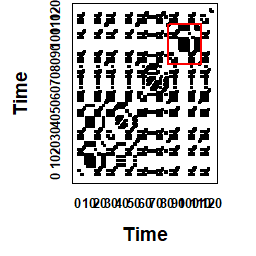## Figure 4 (right panel)

text_zoom = text[81:110]

ans_zoom = crqa(text_zoom, text_zoom, delay, embed, rescale, radius, normalize,
mindiagline, minvertline, tw, whiteline, recpt, side, method, metric,
datatype)
#> Warning in crqa(text_zoom, text_zoom, delay, embed, rescale, radius,
#> normalize, : Your input data was provided either as character or factor, and
#> recoded as numerical identifiers

# Add to the graphics parameters the labels for the axes (x,y)
parC$labay = parC$labax = text_zoom

# Change the las argument to print the words vertically
parC$las = 2 # make it vertical # Change the unit to make it word by word parC$unit = 1 # make it vertical

# Change the label of x, y axis
parC$labelx = parC$labely = "Words"

par(font.lab = 2, font.axis = 2, cex.lab = 2, cex.axis = 1.5)

plotRP(ans_zoom$RP, parC)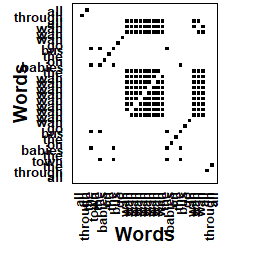## Cross-recurrence quantification analysis (CRQA) listener = eyemovement$listener
narrator = eyemovement$narrator delay = 1; embed = 1; rescale = 0; radius = .01; normalize = 0; mindiagline = 2; minvertline = 2; tw = 0; whiteline = FALSE; recpt = FALSE; side = "both" method = 'crqa'; metric = 'euclidean'; datatype = "categorical" ## Compute cross-recurrence quantification analysis ans = crqa(narrator, listener, delay, embed, rescale, radius, normalize, mindiagline, minvertline, tw, whiteline, recpt, side, method, metric, datatype) ## Uncomment to check performance (but need to load profvis) # pf = profvis(crqa(narrator, listener, delay, embed, rescale, radius, normalize, # mindiagline, minvertline, tw, whiteline, recpt, side, method, metric, # datatype)) RP = as.matrix(ans$RP)
results = unlist(ans[1:10])

## Change again the plotting parameters
parC$labelx = parC$labely = "Time (sec.)"
parC$unit = 333 ## in ms parC$pcex = .1 # make this much smaller as we have loads of points
parC$labax = parC$labay = seq(0, 60, 10)
parC$las = 1 windowstep = 50 ### Figure 5 (left panel) par(font.lab = 2, font.axis = 2, cex.lab = 2, cex.axis = 1.5) plotRP(RP, parC) polygon(xd, yd, col = diagcol, border = F) # the diagonal polygon(xw, yw, col = windcol, border = F) # the first window for (w in 1:10){ # the number of overlapping windows that we want to plot polygon(xw + windowstep, yw + windowstep, col = windcol, border = F) # # the second window polygon(xw + w*windowstep, yw + w*windowstep, col = windcol, border = F) # the third window polygon(xw + w*windowstep, yw + w*windowstep, col = windcol, border = F) # the fourth window }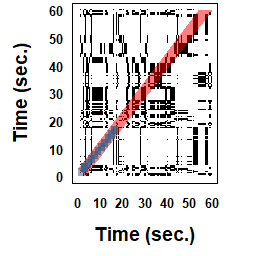### Figure 5 (mid panel) ## Construct the time-course for the diagonal profile timecourse = round( seq(-3300,3300,33)/1000, digit = 2) ## Show diagonal-profile CRQA with eye-movement data res = drpfromts(narrator, listener, windowsize = 100, radius = 0.001, delay = 1, embed = 1, rescale = 0, normalize = 0, mindiagline = 2, minvertline = 2, tw = 0, whiteline = F, recpt = F, side = 'both', method = 'crqa', metric = 'euclidean', datatype = 'continuous') profile = res$profile*100

par(font.lab = 2, font.axis = 2, cex.lab = 1.8, cex.axis = 1.3,
mar = c(4.5, 5.5, 1, 2))

plot(timecourse, profile, type = "l", lwd = 2.5, xlab = "Lag (seconds)",
ylab = "Recurrence Rate %")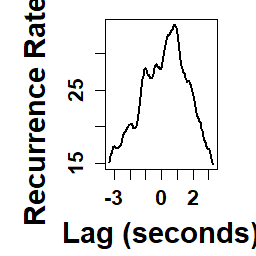### Figure 5 (right panel)

delay = 1; embed = 1; rescale = 1; radius = 0.001;
normalize = 0; mindiagline = 2; minvertline = 2;
tw = 0; whiteline = FALSE; recpt = FALSE; side = "both"
method = 'crqa'; metric = 'euclidean';
datatype = "categorical"; windowsize =  100;
lagwidth = 50; windowstep = 20

## Calculate windowed cross-recurrence
res = windowdrp(narrator, listener, windowstep, windowsize, lagwidth,
radius = 0.001, delay = 1, embed = 1, rescale = 0,
normalize = 0, mindiagline = 2, minvertline = 2,
tw = 0, whiteline = F, side = 'both',
method = 'crqa', metric = 'euclidean',
datatype = "continuous")

profile = res$profile*100 timecourse = round( seq(1, length(profile), 1)*windowstep*.033, digit = 1) par(font.lab = 2, font.axis = 2, cex.lab = 1.8, cex.axis = 1.3, mar = c(4.5, 5.5, 1, 2)) plot(timecourse, profile, type = "l", lwd = 2.5, xlab = "Time (seconds)", ylab = "Recurrence Rate %")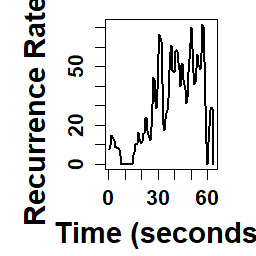### Extra (use wincrqa) listener = eyemovement$listener
narrator = eyemovement$narrator delay = 1; embed = 1; rescale = 0; radius = 0.001; normalize = 0; mindiagline = 2; minvertline = 2; tw = 0; whiteline = FALSE; recpt = FALSE; side = "both" method = 'crqa'; metric = 'euclidean'; datatype = "continuous"; windowsize = 100; windowstep = 20 trend = FALSE ans = wincrqa(listener, narrator, windowstep, windowsize, delay, embed, radius, rescale, normalize, mindiagline, minvertline, tw, whiteline, recpt, side, method, metric, datatype, trend) profile = as.numeric(ans$RR)

plot(profile, type = 'l')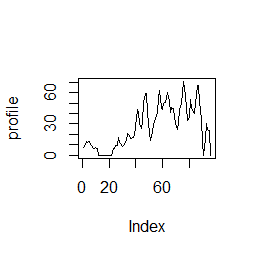## Multidimensional recurrence quantification analysis (MdRQA)

# Reduce the dimensionality of the time series to reduce runtime
# handset = handmovement[1:3000, ]
handset = handmovement[1:1000, ]

P1 = cbind(handset$P1_TT_d, handset$P1_TT_n)
P2 = cbind(handset$P2_TT_d, handset$P2_TT_n)

delay = 5; embed = 2; rescale = 0; radius = .1;
normalize = 0; mindiagline = 10; minvertline = 10;
tw = 0; whiteline = FALSE; recpt = FALSE; side = "both"
method = 'mdcrqa'; metric = 'euclidean';
datatype = "continuous"

ans = crqa(P1, P2, delay, embed, rescale, radius, normalize,
mindiagline, minvertline, tw, whiteline, recpt, side, method, metric,
datatype)

RP = ans$RP results = unlist(ans[1:10]) print(results) #> RR DET NRLINE maxL L ENTR #> 19.6643519 75.4671721 4024.0000000 161.0000000 36.5111829 3.7221108 #> rENTR LAM TT catH #> 0.7408836 83.9759197 23.3335028 NA ## Adjust the plotting parameters parC$unit = 300
parC$pcex = .1 # make this much smaller as we have loads of points parC$labax =  parC$labay = seq(0, nrow(RP), parC$unit)

plotRP(RP, parC)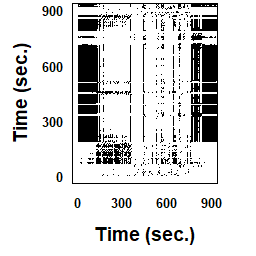## Estimating starting parameters: optimizeParam

## Estimate parameters for uni-dimensional RQA using simulated data generated off sinusoids.
ts1 = seq(0.1, 200, .1)
ts1 = sin(ts1) + linspace(0, 1,length(ts1));
ts2 = ts1

par = list(method = "rqa", metric = "euclidean", maxlag =  20,
rescale = 4, mindiagline = 10, minvertline = 10, tw = 0,
whiteline = FALSE, recpt = FALSE, side = "both",
datatype = "continuous", fnnpercent  = 10,  typeami = 'mindip')

results = optimizeParam(ts1, ts2, par, min.rec = 2, max.rec = 5) # it may take some time
print(unlist(results))
#>  0.1755553  2.0000000 18.0000000

ans = crqa(ts1, ts2, delay = results$delay, embed = results$emddim,
rescale, radius = results$radius) unlist(print(ans[1:10])) #>$RR
#>  2.028753
#>
#> $DET #>  99.39771 #> #>$NRLINE
#>  7127
#>
#> $maxL #>  1982 #> #>$L
#>  11.11492
#>
#> $ENTR #>  2.666816 #> #>$rENTR
#>  0.7181268
#>
#> $LAM #>  97.9861 #> #>$TT
#>  3.205311
#>
#> $catH #>  NA #> RR DET NRLINE maxL L ENTR #> 2.0287532 99.3977113 7127.0000000 1982.0000000 11.1149151 2.6668157 #> rENTR LAM TT catH #> 0.7181268 97.9860972 3.2053113 NA Let optimize parameters of multi-dimensional data using the hand-movements. ## Change a few parameters to set this par$method = "mdcrqa";par$fnnpercent = NA; par$typeami = NA;
par$nbins = 50; par$criterion = "firstBelow"; par$threshold = 1.6; par$maxEmb = 20; par$numSamples = 500; par$Rtol = 10; par$Atol = 2 results = optimizeParam(P1, P2, par, min.rec = 2, max.rec = 5) # it may take some time #> Warning in sqrt((temp[yRd1[j]] - r2d[j])/r2d[j]): NaNs produced #> Warning in sqrt((temp[yRd1[j]] - r2d[j])/r2d[j]): NaNs produced #> Warning in sqrt((temp[yRd1[j]] - r2d[j])/r2d[j]): NaNs produced #> Warning in sqrt((temp[yRd1[j]] - r2d[j])/r2d[j]): NaNs produced #> Warning in sqrt((temp[yRd1[j]] - r2d[j])/r2d[j]): NaNs produced #> Warning in sqrt((temp[yRd1[j]] - r2d[j])/r2d[j]): NaNs produced #> Warning in sqrt((temp[yRd1[j]] - r2d[j])/r2d[j]): NaNs produced #> Warning in sqrt((temp[yRd1[j]] - r2d[j])/r2d[j]): NaNs produced #> Warning in sqrt((temp[yRd1[j]] - r2d[j])/r2d[j]): NaNs produced #> Warning in sqrt((temp[yRd1[j]] - r2d[j])/r2d[j]): NaNs produced #> Warning in sqrt((temp[yRd1[j]] - r2d[j])/r2d[j]): NaNs produced #> Warning in sqrt((temp[yRd1[j]] - r2d[j])/r2d[j]): NaNs produced #> Warning in sqrt((temp[yRd1[j]] - r2d[j])/r2d[j]): NaNs produced print(unlist(results)) #> radius emddim delay #> 0.04152206 4.00000000 2.00000000 ans = crqa(P1, P2, delay = results$delay, embed = results$emddim, rescale, radius = results$radius, normalize,
mindiagline, minvertline, tw, whiteline, recpt, side, method, metric,
datatype)

print(ans[1:10])
#> $RR #>  9.457348 #> #>$DET
#>  80.5687
#>
#> $NRLINE #>  3136 #> #>$maxL
#>  87
#>
#> $L #>  24.0067 #> #>$ENTR
#>  3.417769
#>
#> $rENTR #>  0.7965975 #> #>$LAM
#>  91.88695
#>
#> $TT #>  31.73394 #> #>$catH
#>  NA

### Estimating delay in multidimensional data

nbins = 10; maxlag = 10; criterion = "firstBelow"; threshold = exp(-1)

handset = handmovement[1:300, ] ## take less points

mdDelay(handset, nbins, maxlag, criterion, threshold)
#>  2

### Estimating false nearest neighbours in multidimensional data

tau = 1; maxEmb = 10; numSamples = 500; Rtol = 10; Atol = 2

mdFnn(handset, tau, maxEmb, numSamples, Rtol, Atol)
#> $fnnPerc #>  100.000000 4.123711 2.749141 2.405498 3.780069 4.467354 #>  4.810997 4.123711 4.810997 4.467354 #> #>$embTimes
#>    1  2  3  4  5  6  7  8  9 10

## Breaking down the computation: piecewiseRQA

YLIM  = range(Figure_6$speed) YLIM2 = range(Figure_6$memory)

perfFull  = subset(Figure_6, Figure_6$typeRQA == "full") perfPiece = subset(Figure_6, Figure_6$typeRQA == "piece")

cex = 2.1, xlab = "Number of Data Points", pch = perfPiece$pch, ylab = "Time (sec)", ylim = YLIM, main = "Speed") points(perfFull$datapoint, perfFull$speed, type = "p", col = "black", pch = 19, cex = 2.1)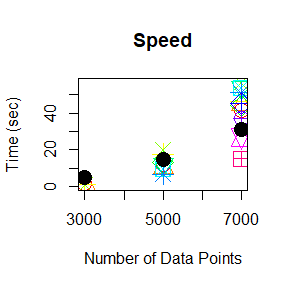### Figure 6 (right panel) plot(perfPiece$datapoint, perfPiece$memory, type = "p", col = perfPiece$colors,
cex = 2.1, xlab = "Number of Data Points", pch = perfPiece$pch, ylab = "Max Memory (Mb)", ylim = YLIM2, main = "Memory") points(perfFull$datapoint, perfFull$memory, type = "p", col = "black", pch = 19, cex = 2.1) legend("topleft",legend = c(unique(perfPiece$blocksize), "full"),
col = c(unique(perfPiece$colors), "black"), cex = 1.5, pch = c(unique(perfPiece$pch), 19), bty = "n")